# Pi

Jump to: navigation, search

Pi, or Archimedes' constant, is perhaps the most important mathematical constant in all of mathematics and engineering. It is defined as the ratio of the circumference of a circle to its diameter, and represented by the 16th letter of the Greek alphabet,. It was first used with its current meaning in 1706 by a Welsh mathematician William Jones, who selectedbecause it is the first letter of the Greek word for perimeter (περίμετρος), i.e. the circumference of a circle is its perimeter. A Swiss mathematician, Leonhard Euler, brought the concept into general use in 1737.is referenced in the Bible, literature, and even as the title of a movie. It is central to the most famous identity in all of mathematics: Euler's Identity.

The value ofis approximately 3.1416, or 22/7. The exact value cannot be expressed as a fraction or as a decimal number, regardless of how many digits are used. Johann Heinrich Lambert proved this in 1761 by showing thatis an irrational number, which means that it can't be expressed as a fraction. In 1882, Carl Louis Ferdinand von Lindeman proved thatis also a transcendental number, which means that it can't be expressed as the solution to any simple equation.

## History

Mathematicians have worked for centuries to calculateto more and more decimal places. To some extent, the progress of mathematics, or at least of computation, can be gauged by the progress in the number of digits to whichhas been calculated.

Some ancients expressedby using fractional approximations. The Rhind, or Ahmes Papyrus (c. 1650 B.C.) has shown that the ancient Egyptians had determined the value forto be 3.1605. The Babylonian value from the same era was 3 1/8 = 3.125., coming to within 1 percent accuracy for both.

Archimedes of Syracuse (287-212 BC) carried out "the first theoretical calculation" of., using regular polygons with a total of 96 sides, within and circumscribing a circle, and in about 225 B.C. he came up with a formula between the folowing numbers: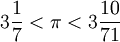This is ten times better than the Egyptian and Babylonian values, and it is within 0.04 percent of the correct value. The German-Dutch mathematician Ludolph Van Ceulen used Archimedes's formula to calculateto 32 digits in 1615. He was so proud of his achievement that he had the digits engraved on his tombstone.

Towards the end of the 17th century mathematical analysis had new methods of making calculations, andwas no exception. Brilliant mathematicians had made individual calculations forin a variety of ways. In 1593 François Viète (1540-1603) wrote the expression: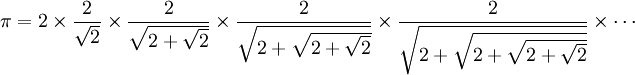John Wallis (1616-1703) made his expression 1655, proving that: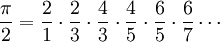William Brouncker (1620-1684) discovered the continued fraction in 1658:During the plague of 1665-1666, Isaac Newton was confined to the English village of Woolsthorpe. There he calculatedto 16 digits.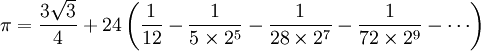Unlike Van Ceulen, Newton did not consider his calculations to be an achievement. "I am ashamed to tell you to how many figures I carried these computations, having no other business at the time," he wrote.

Gottfried Wilhelm von Leibniz (1646-1716) published a series forin 1673, coming up with the formulas: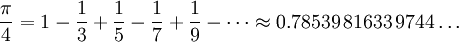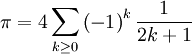Brouncker's convergents are related to the Leibniz formula for pi: for instance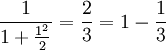andIn 1706, John Machin, secretary of England's Royal Society, developed a quickly converging formula forand used it to calculated the first 100 digits. In 1844, the idiot savant Johann Dase of Hamburg used Machin's formula to calculate 200 digits in less than two months. In contrast, William Shanks spent twenty years calculatingto 707 places, a task he completed in 1873. In 1945, it was discovered that only the first 527 of Shanks's digits were correct.

ENIAC, the first electronic computer, took seventy hours to calculate 2,037 digits in 1949. In 2008, the first million digits ofwere published on Project Gutenberg. In 2014, the anonymous programmer Houkouonchi calculated the first 13.3 trillion digits ofin 208 days. This result has not been published.

##in mathematics

It's impossible to overestimate the importance of(and e) for mathematics. These values are tied by Euler's identity:.may be used to determine the area of a circle:## Recreation

Memorizingis a challenge that appeals to some people. Mnemonics have been devised. Counting the letters in each word of the phrase "Now I want a drink—alcoholic, of course" givesto seven places (which is more than enough for all ordinary purposes). Numerous other mnemonics of this kind have been devised; in 1995, Michael Keith wrote one entitled Near a Raven which simultaneously parodies Edgar Allen Poe's poem The Raven, while encodingto 740 places.

March 14 marks Pi Day, a holiday on which the mathematical constant is celebrated. The date, 3/14, comes from the first three digits of. Some people begin their celebration at 1:59 pm, derived from the following three digits.

Pi Approximation Day is a similar holiday, celebrated on 22 July (from the approximation 22/7).

The value of pi is approximately:

3.14159265358979323846264338327950288419​7169399375​1058209749​4459230781​6406286208​9986280348​2534211706​7982148086​5132823066​4709384460​9550582231​7253594081​2848111745​0284102701​9385211055​5964462294​8954930381​9644288109​7566593344​6128475648​2337867831​6527120190​9145648566​92...

## Does the Bible attempt to define pi?

Virtually all serious students of the Bible say no. Still, critics frequently claim that the Bible contains an incorrect value for, and the question is raised frequently enough to earn mention in the Skeptics Annotated Bible.

The claim is based on a verse in the first book of Kings:

He made the Sea of cast metal, circular in shape, measuring ten cubits from rim to rim and five cubits high. It took a line of thirty cubits to measure around it. 1_Kings 7:23 (NIV)

Thus, critics say, the Bible claims that the value ofis three, and is therefore in error. However, there are a number of assumptions in this claim, any of which might invalidate the argument if wrong:
• That the tools and system of measurement available to the builders were sufficiently accurate to distinguish between three and.
• That the phrase translated as "circular in shape" means perfectly circular, not simply "round" as an ellipse is round.
• That the Bible is trying to provide a value for, and not merely describing the historical event of building this object.
• That a value is wrong simply because it depends on imprecisely expressed divisors. The ratio that iscan be derived completely accurately for a whole range of values even if the Sea is taken as a perfect circle, namely when, before rounding to the nearest whole number, the diameter is known to be greater than 9.5 and less than about 9.708, and the circumference greater than about 29.845 and less than 30.5, it being quite common at the time to round to whole numbers.
• That both the diameter and the circumference are measuring the same edges. Since the sides of any practical vessel have thickness, it is possible that the diameter is an outside measurement and the circumference is an inside measurement.
• That both the diameter and the circumference are measuring the same part of the object. The object is also described as having an outward-turned rim. The easiest places to measure the diameter would be across the wider rim, and the easiest place to measure the circumference would be around the body below the rim.

Common sense and a rudimentary knowledge of the Bible should cause one to question whether it sets out to define mathematical concepts. The creation of a "sea of cast metal" by human beings in ancient times, without modern construction tools and measuring equipment, does not require nor could it utilize a precise value for. An even more fundamental objection is thatis an irrational number, and therefore has an infinite number of digits. (A "closed form" ofdoes exist, but requires mathematical notation that was invented many centuries later.) A decimal expression ofcould not "fit" in the Bible, or in any other finite text.